Next: 3.2 Mathematical Formulation Up: 3. Advanced Oxidation Model Previous: 3. Advanced Oxidation Model

# 3.1 The Diffuse Interface Concept

The diffuse interface concept avoids a moving interface problem, because there is not a sharp interface between silicon and SiO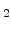in contrast to the standard models [64,65]. Because of the missing sharp interface there different segments for silicon and SiOdo not exist. In order to determine where is silicon and where is SiO, a parameter named normalized silicon is defined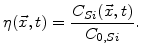(3.1)

Here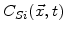is the silicon concentration at time t and point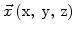and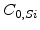is the concentration in pure silicon.is 1 in pure silicon and 0 in pure silicon dioxide.

Instead of a sharp interface there is a so-called reaction layer where the diffusion of oxidants, the chemical reaction, and the volume increase occur simultaneously. This reaction layer has a spatial finite width (see Fig. 3.1), where the values oflie between 0 and 1 . Thecurve always starts with 0 near silicon and ends at 1 near oxide, as shown in Fig. 3.2. The shape of this curve is given by the calculateddistribution in the reaction layer, which depends on the parameters in the model.Next: 3.2 Mathematical Formulation Up: 3. Advanced Oxidation Model Previous: 3. Advanced Oxidation Model

Ch. Hollauer: Modeling of Thermal Oxidation and Stress Effects